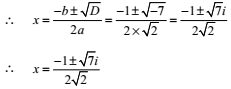## NCERT Solutions for Class 11 Maths Chapter 5 Complex Numbers and Quadratic Equations Exercise 5.3

Chapter 5 Complex Numbers and Quadratic Equations Exercise 5.3 is available here that will help you in solving any difficult questions present in the exercise and also for framing your own answers. Class 11 Maths NCERT Solutions given here are prepared by team of Studyrankers subject experts who have taken every care to detail every answer so students can understand the concepts easily.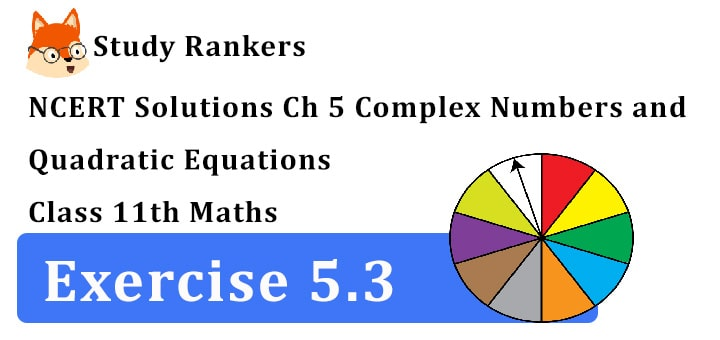1. Solve each of the following equations:
x2 + 3 = 0

x2 + 3 = 0 ∴ x2 = -3
∴ x = ±√-3 = ±√3i

2. 2x2 + x + 1 = 0

2x2 + x + 1 = 0 comparing with ax2 + bx + c = 0
a = 2, b = 1, c = 1
b2 – 4ac = 12 – 4.2.1 = 1 – 8 = -7
∴ x = (-b ± √b2-4ac )/2a = (-1 ± √-7)/2.2
= (-1 ± √7i)/4

3. x2 + 3x + 9 = 04. -x2 + x – 2 = 0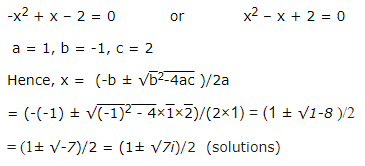5. x2 + 3x + 5 = 0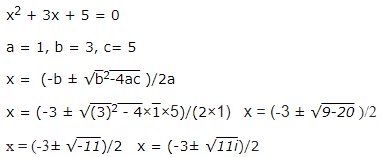6. x2 – x + 2 = 0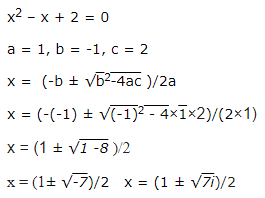7. √2x2 + x + √2 = 08. √3x2 – √2x + 3√3 = 0

We have a = √3, b = -√2, c = 3√3
∴ Discriminant of the equations is D = b2 – 4
ac = (-√2)2 – 4×√3×3√3
=> D = 2 – 36 = -34
∴ x = (-b ± √D)/2a = (√2 ± √-34)/2×√3 = (√2 ± √34i)/2√3
∴ x = (√2 ± √34i)/2√3

9. x2 + x + 1/√2 = 010. x2 + x/√2 + 1 = 0